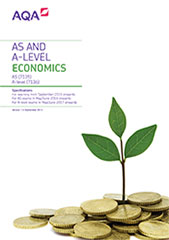# Annex: Quantitative skills in Economics

## AS

In order to develop their skills, knowledge and understanding in economics, students need to have acquired competence in the quantitative skills that are relevant to the subject content and which are applied in the context of an economics AS, including:

• calculate, use and understand ratios and fractions
• calculate, use and understand percentages and percentage changes
• understand and use the terms mean, median
• construct and interpret a range of standard graphical forms
• calculate and interpret index numbers
• calculate cost, revenue and profit (average, totals)
• make calculations to convert from money to real terms
• make calculations of elasticity and interpret the result
• interpret, apply and analyse information in written, graphical and numerical forms

The assessment of quantitative skills will include at least Level 2 mathematical skills as a minimum of 15% of the overall AS marks. These skills may be assessed across the assessment objectives.

## A-level

In order to develop their skills, knowledge and understanding in economics, students need to have acquired competence in the quantitative skills that are relevant to the subject content and which are applied in the context of an economics A-level, including:

• calculate, use and understand ratios and fractions
• calculate, use and understand percentages and percentage changes
• understand and use the terms mean, median and relevant quantiles
• construct and interpret a range of standard graphical forms
• calculate and interpret index numbers
• calculate cost, revenue and profit (marginal, average, totals)
• make calculations to convert from money to real terms
• make calculations of elasticity and interpret the result
• interpret, apply and analyse information in written, graphical and numerical forms.

The assessment of quantitative skills will include at least Level 2 mathematical skills as a minimum of 20% of the overall A-level marks. These skills may be assessed across the assessment objectives.